prev next
 File: /Characters/UTF8/m.xml Author: MathML 2 Recommendation Description: Wide Characters starting with m Sample Rendering: N/A

Your browser's rendering:

namepicrender
macr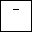$¯$
male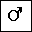$♂$
malt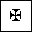$✠$
maltese$✠$
map$↦$
Map$⤅$
mapsto$↦$
mapstodown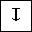$↧$
mapstoleft$↤$
mapstoup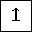$↥$
marker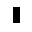$▮$
mcomma$⨩$
Mcy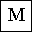$М$
mcy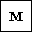$м$
mdash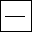$—$
mDDot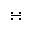$∺$
measuredangle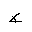$\measuredangle$
MediumSpace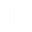Mellintrf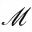$ℳ$
Mfr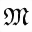$𝔐$
mfr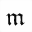$𝔪$
mho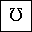$\mho$
micro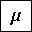$µ$
mid$\mid$
midast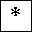$*$
midcir$⫰$
middot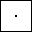$·$
minus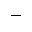$-$
minusb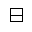$\boxminus$
minusd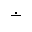$\dot{-}$
minusdu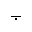$⨪$
MinusPlus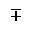$\mp$
mlcp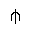$⫛$
mldr$\dots$
mnplus$\mp$
models$\vDash$
Mopf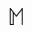$𝕄$
mopf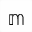$𝕞$
mp$\mp$
mscr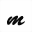$𝓂$
Mscr$ℳ$
mstpos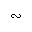$∾$
mu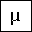$\mu$
multimap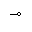$⊸$
mumap$⊸$

Source Code:

```        N/A
```